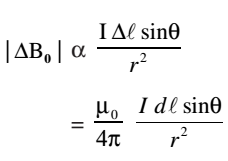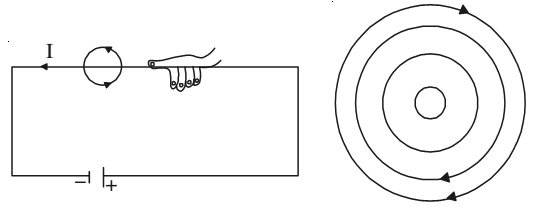# Biot-Savart’s Law

Biot-Savart’s law gives a quantitative relationship between current in conductor and the resulting magnetic field at a point in the space around it. Each part of a current carrying conductor contributes to magnetic field around it. The net value of B at a point is thus the combined effect of all the individual parts of the conductor.

The net magnetic field due to any current carrying conductor is the vector sum of the contributions due to the current in each infinitesimal element of length ∆L. Experiments show that the field B due to an element ∆L depends on

• current flowing through the conductor, I
• length of the element ∆L
• inversely proportional to the square of the distance of observation point P from the element ∆L
• the angle between the element and the line joining the element to the observation pointwhere µ0 is permeability of vacuum. Its value is 4π×10–7 WA–1m–1. The value of permeability of air is also nearly equal to µ0. If the conductor is placed in a medium other than air, the value of the field is altered and is given by |B| = µ0|B|. Here, µ represents the permeability of the medium.

### Direction of B

Magnetic field at a point is a vector quantity. The direction of B may be determined by applying the right hand grip rule

Grasp the wire in your right hand so that the thumb points in the direction of the current. Then the curled fingers of the hand will point in the direction of the magnetic field. To represent the magnetic field on paper, consider that current is flowing into the plane of the paper. Then according to the right hand rule, the field lines shall be in the plane of the paper.